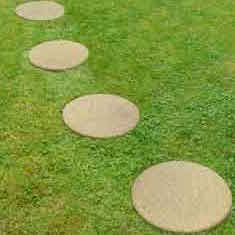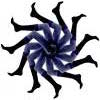(STEP1) Choose any two numbers from the 7 times table. Add them together. What do you notice? Repeat with some other examples, always choosing pairs of numbers from the same times table. What do you notice? Does the same thing always happen? Why or why not?

(STEP 2) Choose two digits and arrange them to make two double-digit numbers. For example if you choose 5 and 2, you can make 52 and 25. Now add your two-digit numbers. Repeat with some other examples. What do you notice? Does the same thing always happen? Why or why not?

(STEP 3) Look at this sequence of numbers: 11, 101, 1001, 10001, 100001, …
Can you describe the sequence?
Divide numbers in this sequence by 11, WITHOUT using a calculator.
What do you notice? Does the same thing always happen? Why or why not?

(STEP 4) Take any four-digit number, move the first digit to the ‘back of the queue’ and move the rest along. For example 5238 would become 2385.

Is the answer always a multiple of 11? Can you prove it?

What can you say about how this process works?

As a bigger challenge: What happens when you do this with three-digit numbers? Five-digit numbers? Six-digit numbers?

Try some examples.

When does this process give a multiple of 11 and when is it not a multiple of 11?